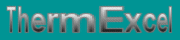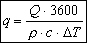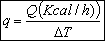Unit of energy (joule, calorie), unit of power. Calculation of the water flow for the thermal transfer.
French site
| Home | Thematic | Tables | Programs | Library | Download | Links | Contact | Forum |--

Thermal fluid flow
 Thematic Quotation Margin Hydraulic Linear pressure loss Local pressure loss Compressible fluids No circular duct Thermal Thermal fluid flow Pipe sizing steam Control valves Orifice plate Pumps Ventilators Gas Fuel gasEnergy

The legal unit of energy (or work) in the metric system is the Joule (j)

Use of the Calorie
The use of the calorie ceased on December 31, 1977 officially.

Contrary to the Joule, the calorie which is still used is quantified under various values.

• The general standard, it is the quantity of heat necessary to raise 1°C the temperature of 1 gram of water of 14.5 to 15.5°C under the normal atmospheric pressure.
• 1 calorie = 4.186 J - 0.003968 Btu - 3.087 foot pounds
• 1 kg of water with 15°C under 1 atm = 4.186 kj/kg °C or 1 kcal/kg °C
• 1 kcal = 1.163 W

The small calorie or therm, is measured 3.5°C with 4.5°C (water is densest at 4°C); The Calorie, is the equivalent with 1,000 small calories.

The intermediate value of the calorie in the range of 0°C with 100°C is called the average calorie; i.e. 1/100 of energy necessary to heat the water of 1 gram of water of the melting point at its point of boiling.

Two other calories are sometimes used in "International Steam Table calorie" equivalent to 4.187J (1.163 W) and the "Thermo chemical calorie" equivalent to 4.184 J (1.1622 W)

 Power units

The power is the unit of energy per unit of time.

Unit of legal power in the metric system is the Watt (w)

• 1 Joule is equal to 1 Watt during one second.
• Joule (j) = 1 w/s or Newton meter (Nm)
• 1 watt/h = 3600 Joules
 Calculation of the flow for the thermal transfer

The flow of the thermal fluid is determined by the following formula:With:

• q = Water flow in m3/h
• Q = Thermal power to convey in w/h
• r = Density of water on the circuit in kg/m3
• c = Specific heat of water in kJj/kg °k
• DT = Temperature in °K.

The temperature of reference taken into account in the elements of pressure losses is established on the average temperature between the inlet and the outlet and thus in consequence of the thermal type of distribution (hot Water or cooling water)

Annotation

The water flow necessary for the thermal transfer is usually determined by the following usual formula:In this case more the temperature will be high and more the margin of error will be consequent.

Example:

116264 w/h (that is to say 100000 Kcal/h) to transfer in a distribution system of water at 90 / 70°K under a pressure of 2.5 bar (values usually adopted in the heating installations)

The water flow usually calculated will be of 5000 l/h

Actually:

• Density of water at 80°C and 2.5 bar = 971.6 kg/m3
• Specific heat of water at 80°C and 2.5 bars = 4.196 j/kg °C
• The actual water flow to make forward will be 5133 liters
• The margin of error is 2,66%

Last update: